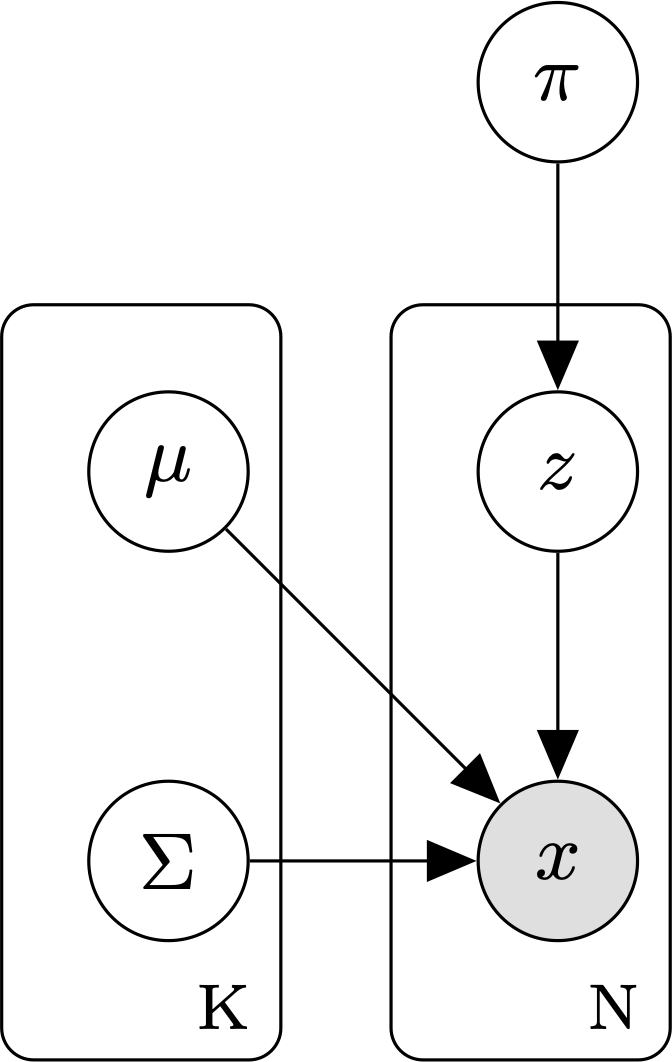# Gaussian mixture models¶

A Gaussian mixture model (GMM) is a latent variable model commonly used for unsupervised clustering.Graphical model for a GMM with K mixture components and N data points.

A GMM assumes that:

1. The observed data are generated from a mixture distribution, P, made up of K mixture components.
2. Each mixture component is a multivariate Gaussian with its own mean $$\mu$$, covariance matrix, $$\Sigma$$, and mixture weight, $$\pi$$.

The parameters of a GMM model are:

• $$\theta$$, the set of parameters for each of the K mixture components. $$\theta = \{ \mu_1, \Sigma_1, \pi_i, \ldots, \mu_k, \Sigma_k, \pi_k \}$$.

Under a GMM, the joint probability of a sequence of cluster assignments Z and an observed dataset $$X = \{x_1, \ldots, x_N \}$$, is:

$p(Z, X \mid \theta) = \prod_{i=1}^N p(z_i, x_i \mid \theta) = \prod_{i=1}^N \prod_{k=1}^K [\mathcal{N}(x_i \mid \mu_k, \Sigma_k) \pi_k ]^{\mathbb{1}_{[z_{i} = k]}}$

where

• $$\theta$$ is the set of GMM parameters: $$\theta = \{ \mu_1, \Sigma_1, \pi_i, \ldots, \mu_k, \Sigma_k, \pi_k \}$$.
• $$Z_i \in \{ 1, \ldots, k \}$$ is a latent variable reflecting the ID of the mixture component that generated data point i.
• $$\mathbb{1}_{[z_i = k]}$$ is a binary indicator function returning 1 if data point $$x_i$$ was sampled from mixture component $$k$$ and 0 otherwise.

As with other latent-variable models, we use the expectation-maximization (EM) algorithm to learn the GMM parameters.

Models

References

  Bilmes, J. A. (1998). “A gentle tutorial of the EM algorithm and its application to parameter estimation for Gaussian mixture and hidden Markov models” International Computer Science Institute, 4(510) https://www.inf.ed.ac.uk/teaching/courses/pmr/docs/EM.pdf